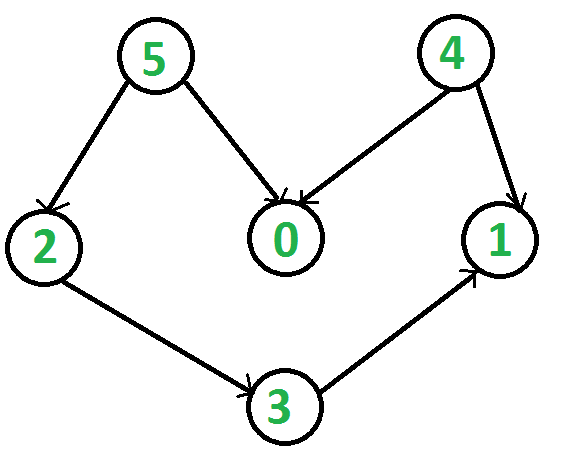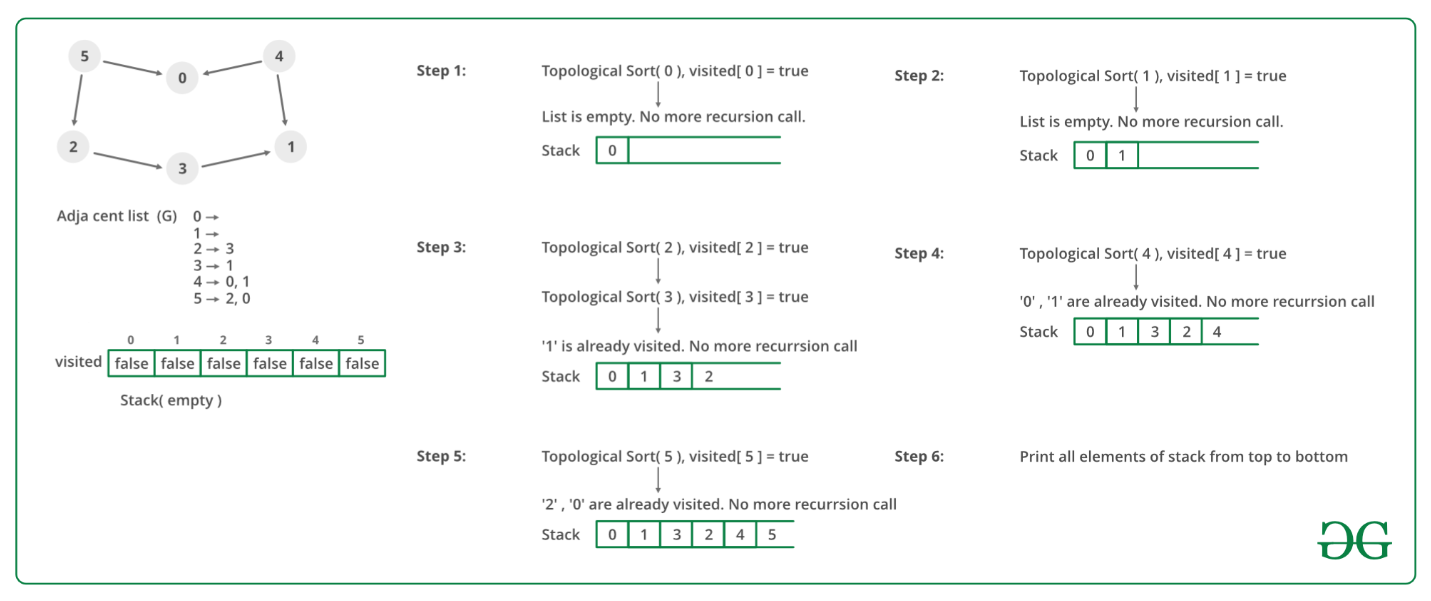# Topological Sorting

• Difficulty Level : Medium
• Last Updated : 05 Mar, 2021

Topological sorting for Directed Acyclic Graph (DAG) is a linear ordering of vertices such that for every directed edge u v, vertex u comes before v in the ordering. Topological Sorting for a graph is not possible if the graph is not a DAG.

For example, a topological sorting of the following graph is “5 4 2 3 1 0”. There can be more than one topological sorting for a graph. For example, another topological sorting of the following graph is “4 5 2 3 1 0”. The first vertex in topological sorting is always a vertex with in-degree as 0 (a vertex with no incoming edges).

Become a success story instead of just reading about them. Prepare for coding interviews at Amazon and other top product-based companies with our Amazon Test Series. Includes topic-wise practice questions on all important DSA topics along with 10 practice contests of 2 hours each. Designed by industry experts that will surely help you practice and sharpen your programming skills. Wait no more, start your preparation today!Topological Sorting vs Depth First Traversal (DFS)

In DFS, we print a vertex and then recursively call DFS for its adjacent vertices. In topological sorting, we need to print a vertex before its adjacent vertices. For example, in the given graph, the vertex ‘5’ should be printed before vertex ‘0’, but unlike DFS, the vertex ‘4’ should also be printed before vertex ‘0’. So Topological sorting is different from DFS. For example, a DFS of the shown graph is “5 2 3 1 0 4”, but it is not a topological sorting.

Algorithm to find Topological Sorting:

We recommend to first see the implementation of DFS. We can modify DFS to find Topological Sorting of a graph. In DFS, we start from a vertex, we first print it and then recursively call DFS for its adjacent vertices. In topological sorting, we use a temporary stack. We don’t print the vertex immediately, we first recursively call topological sorting for all its adjacent vertices, then push it to a stack. Finally, print contents of the stack. Note that a vertex is pushed to stack only when all of its adjacent vertices (and their adjacent vertices and so on) are already in the stack.

Below image is an illustration of the above approach:Following are the implementations of topological sorting. Please see the code for Depth First Traversal for a disconnected Graph and note the differences between the second code given there and the below code.

## C++

 `// A C++ program to print topological``// sorting of a DAG``#include ``#include ``#include ``using` `namespace` `std;` `// Class to represent a graph``class` `Graph {``    ``// No. of vertices'``    ``int` `V;` `    ``// Pointer to an array containing adjacency listsList``    ``list<``int``>* adj;` `    ``// A function used by topologicalSort``    ``void` `topologicalSortUtil(``int` `v, ``bool` `visited[],``                             ``stack<``int``>& Stack);` `public``:``    ``// Constructor``    ``Graph(``int` `V);` `    ``// function to add an edge to graph``    ``void` `addEdge(``int` `v, ``int` `w);` `    ``// prints a Topological Sort of``    ``// the complete graph``    ``void` `topologicalSort();``};` `Graph::Graph(``int` `V)``{``    ``this``->V = V;``    ``adj = ``new` `list<``int``>[V];``}` `void` `Graph::addEdge(``int` `v, ``int` `w)``{``    ``// Add w to v’s list.``    ``adj[v].push_back(w);``}` `// A recursive function used by topologicalSort``void` `Graph::topologicalSortUtil(``int` `v, ``bool` `visited[],``                                ``stack<``int``>& Stack)``{``    ``// Mark the current node as visited.``    ``visited[v] = ``true``;` `    ``// Recur for all the vertices``    ``// adjacent to this vertex``    ``list<``int``>::iterator i;``    ``for` `(i = adj[v].begin(); i != adj[v].end(); ++i)``        ``if` `(!visited[*i])``            ``topologicalSortUtil(*i, visited, Stack);` `    ``// Push current vertex to stack``    ``// which stores result``    ``Stack.push(v);``}` `// The function to do Topological Sort.``// It uses recursive topologicalSortUtil()``void` `Graph::topologicalSort()``{``    ``stack<``int``> Stack;` `    ``// Mark all the vertices as not visited``    ``bool``* visited = ``new` `bool``[V];``    ``for` `(``int` `i = 0; i < V; i++)``        ``visited[i] = ``false``;` `    ``// Call the recursive helper function``    ``// to store Topological``    ``// Sort starting from all``    ``// vertices one by one``    ``for` `(``int` `i = 0; i < V; i++)``        ``if` `(visited[i] == ``false``)``            ``topologicalSortUtil(i, visited, Stack);` `    ``// Print contents of stack``    ``while` `(Stack.empty() == ``false``) {``        ``cout << Stack.top() << ``" "``;``        ``Stack.pop();``    ``}``}` `// Driver Code``int` `main()``{``    ``// Create a graph given in the above diagram``    ``Graph g(6);``    ``g.addEdge(5, 2);``    ``g.addEdge(5, 0);``    ``g.addEdge(4, 0);``    ``g.addEdge(4, 1);``    ``g.addEdge(2, 3);``    ``g.addEdge(3, 1);` `    ``cout << ``"Following is a Topological Sort of the given "``            ``"graph \n"``;` `    ``// Function Call``    ``g.topologicalSort();` `    ``return` `0;``}`

## Java

 `// A Java program to print topological``// sorting of a DAG``import` `java.io.*;``import` `java.util.*;` `// This class represents a directed graph``// using adjacency list representation``class` `Graph {``    ``// No. of vertices``    ``private` `int` `V;` `    ``// Adjacency List as ArrayList of ArrayList's``    ``private` `ArrayList > adj;` `    ``// Constructor``    ``Graph(``int` `v)``    ``{``        ``V = v;``        ``adj = ``new` `ArrayList >(v);``        ``for` `(``int` `i = ``0``; i < v; ++i)``            ``adj.add(``new` `ArrayList());``    ``}` `    ``// Function to add an edge into the graph``    ``void` `addEdge(``int` `v, ``int` `w) { adj.get(v).add(w); }` `    ``// A recursive function used by topologicalSort``    ``void` `topologicalSortUtil(``int` `v, ``boolean` `visited[],``                             ``Stack stack)``    ``{``        ``// Mark the current node as visited.``        ``visited[v] = ``true``;``        ``Integer i;` `        ``// Recur for all the vertices adjacent``        ``// to thisvertex``        ``Iterator it = adj.get(v).iterator();``        ``while` `(it.hasNext()) {``            ``i = it.next();``            ``if` `(!visited[i])``                ``topologicalSortUtil(i, visited, stack);``        ``}` `        ``// Push current vertex to stack``        ``// which stores result``        ``stack.push(``new` `Integer(v));``    ``}` `    ``// The function to do Topological Sort.``    ``// It uses recursive topologicalSortUtil()``    ``void` `topologicalSort()``    ``{``        ``Stack stack = ``new` `Stack();` `        ``// Mark all the vertices as not visited``        ``boolean` `visited[] = ``new` `boolean``[V];``        ``for` `(``int` `i = ``0``; i < V; i++)``            ``visited[i] = ``false``;` `        ``// Call the recursive helper``        ``// function to store``        ``// Topological Sort starting``        ``// from all vertices one by one``        ``for` `(``int` `i = ``0``; i < V; i++)``            ``if` `(visited[i] == ``false``)``                ``topologicalSortUtil(i, visited, stack);` `        ``// Print contents of stack``        ``while` `(stack.empty() == ``false``)``            ``System.out.print(stack.pop() + ``" "``);``    ``}` `    ``// Driver code``    ``public` `static` `void` `main(String args[])``    ``{``        ``// Create a graph given in the above diagram``        ``Graph g = ``new` `Graph(``6``);``        ``g.addEdge(``5``, ``2``);``        ``g.addEdge(``5``, ``0``);``        ``g.addEdge(``4``, ``0``);``        ``g.addEdge(``4``, ``1``);``        ``g.addEdge(``2``, ``3``);``        ``g.addEdge(``3``, ``1``);` `        ``System.out.println(``"Following is a Topological "``                           ``+ ``"sort of the given graph"``);``        ``// Function Call``        ``g.topologicalSort();``    ``}``}``// This code is contributed by Aakash Hasija`

## Python

 `# Python program to print topological sorting of a DAG``from` `collections ``import` `defaultdict` `# Class to represent a graph`  `class` `Graph:``    ``def` `__init__(``self``, vertices):``        ``self``.graph ``=` `defaultdict(``list``)  ``# dictionary containing adjacency List``        ``self``.V ``=` `vertices  ``# No. of vertices` `    ``# function to add an edge to graph``    ``def` `addEdge(``self``, u, v):``        ``self``.graph[u].append(v)` `    ``# A recursive function used by topologicalSort``    ``def` `topologicalSortUtil(``self``, v, visited, stack):` `        ``# Mark the current node as visited.``        ``visited[v] ``=` `True` `        ``# Recur for all the vertices adjacent to this vertex``        ``for` `i ``in` `self``.graph[v]:``            ``if` `visited[i] ``=``=` `False``:``                ``self``.topologicalSortUtil(i, visited, stack)` `        ``# Push current vertex to stack which stores result``        ``stack.append(v)` `    ``# The function to do Topological Sort. It uses recursive``    ``# topologicalSortUtil()``    ``def` `topologicalSort(``self``):``        ``# Mark all the vertices as not visited``        ``visited ``=` `[``False``]``*``self``.V``        ``stack ``=` `[]` `        ``# Call the recursive helper function to store Topological``        ``# Sort starting from all vertices one by one``        ``for` `i ``in` `range``(``self``.V):``            ``if` `visited[i] ``=``=` `False``:``                ``self``.topologicalSortUtil(i, visited, stack)` `        ``# Print contents of the stack``        ``print``(stack[::``-``1``])  ``# return list in reverse order`  `# Driver Code``g ``=` `Graph(``6``)``g.addEdge(``5``, ``2``)``g.addEdge(``5``, ``0``)``g.addEdge(``4``, ``0``)``g.addEdge(``4``, ``1``)``g.addEdge(``2``, ``3``)``g.addEdge(``3``, ``1``)` `print` `(``"Following is a Topological Sort of the given graph"``)` `# Function Call``g.topologicalSort()` `# This code is contributed by Neelam Yadav`

## C#

 `// A C# program to print topological``// sorting of a DAG``using` `System;``using` `System.Collections.Generic;` `// This class represents a directed graph``// using adjacency list representation``class` `Graph {` `    ``// No. of vertices``    ``private` `int` `V;` `    ``// Adjacency List as ArrayList``    ``// of ArrayList's``    ``private` `List > adj;` `    ``// Constructor``    ``Graph(``int` `v)``    ``{``        ``V = v;``        ``adj = ``new` `List >(v);``        ``for` `(``int` `i = 0; i < v; i++)``            ``adj.Add(``new` `List<``int``>());``    ``}` `    ``// Function to add an edge into the graph``    ``public` `void` `AddEdge(``int` `v, ``int` `w) { adj[v].Add(w); }` `    ``// A recursive function used by topologicalSort``    ``void` `TopologicalSortUtil(``int` `v, ``bool``[] visited,``                             ``Stack<``int``> stack)``    ``{` `        ``// Mark the current node as visited.``        ``visited[v] = ``true``;` `        ``// Recur for all the vertices``        ``// adjacent to this vertex``        ``foreach``(``var` `vertex ``in` `adj[v])``        ``{``            ``if` `(!visited[vertex])``                ``TopologicalSortUtil(vertex, visited, stack);``        ``}` `        ``// Push current vertex to``        ``// stack which stores result``        ``stack.Push(v);``    ``}` `    ``// The function to do Topological Sort.``    ``// It uses recursive topologicalSortUtil()``    ``void` `TopologicalSort()``    ``{``        ``Stack<``int``> stack = ``new` `Stack<``int``>();` `        ``// Mark all the vertices as not visited``        ``var` `visited = ``new` `bool``[V];` `        ``// Call the recursive helper function``        ``// to store Topological Sort starting``        ``// from all vertices one by one``        ``for` `(``int` `i = 0; i < V; i++) {``            ``if` `(visited[i] == ``false``)``                ``TopologicalSortUtil(i, visited, stack);``        ``}` `        ``// Print contents of stack``        ``foreach``(``var` `vertex ``in` `stack)``        ``{``            ``Console.Write(vertex + ``" "``);``        ``}``    ``}` `    ``// Driver code``    ``public` `static` `void` `Main(``string``[] args)``    ``{` `        ``// Create a graph given``        ``// in the above diagram``        ``Graph g = ``new` `Graph(6);``        ``g.AddEdge(5, 2);``        ``g.AddEdge(5, 0);``        ``g.AddEdge(4, 0);``        ``g.AddEdge(4, 1);``        ``g.AddEdge(2, 3);``        ``g.AddEdge(3, 1);` `        ``Console.WriteLine(``"Following is a Topological "``                          ``+ ``"sort of the given graph"``);` `        ``// Function Call``        ``g.TopologicalSort();``    ``}``}` `// This code is contributed by Abhinav Galodha`
Output
```Following is a Topological Sort of the given graph
5 4 2 3 1 0 ```

Complexity Analysis:

• Time Complexity: O(V+E).
The above algorithm is simply DFS with an extra stack. So time complexity is the same as DFS which is.
• Auxiliary space: O(V).
The extra space is needed for the stack.

Note: Here, we can also use vector instead of the stack. If the vector is used then print the elements in reverse order to get the topological sorting.

Applications:
Topological Sorting is mainly used for scheduling jobs from the given dependencies among jobs. In computer science, applications of this type arise in instruction scheduling, ordering of formula cell evaluation when recomputing formula values in spreadsheets, logic synthesis, determining the order of compilation tasks to perform in make files, data serialization, and resolving symbol dependencies in linkers .

Related Articles:
Kahn’s algorithm for Topological Sorting : Another O(V + E) algorithm.
All Topological Sorts of a Directed Acyclic Graph

References:
http://www.personal.kent.edu/~rmuhamma/Algorithms/MyAlgorithms/GraphAlgor/topoSort.htm
http://en.wikipedia.org/wiki/Topological_sorting